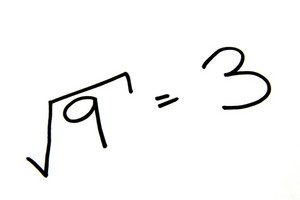Worksheets and No Prep Teaching Resources
Math Worksheets

# Square Roots Worksheets

Help students know that square roots are not for squares with these cool worksheets that explore perfect squares, factoring, completing the square, and more. Since square roots appear all the time in higher math, these worksheets will make sure students are fluent in this vital math topic and that they have a good time, too.Area and Circumference of a Circle
Perfect Squares and Square Roots
Write each perfect square, using the power 2
Write each perfect square as a square root
Find the square root
Find the value of n
Estimate a square root (multiple choice)

Square Root Day

Polynomial Worksheets
Factoring: Perfect-Square Trinomials
Factoring: Perfect-Squares (more difficult)